# Top-10 Visual Reasoning Questions For CMAT & TISSNET PDF

0
614

## Top-10 Visual Reasoning Questions For CMAT & TISSNET

Download CMAT and TISSNET Visual Reasoning Questions and Answers PDF covering the important questions. Most expected Abstract Reasoning (LR) questions with explanations for CMAT & TISSNET 2021 exams.

Question 1: Choose the mirror image for the following figure:

a) A

b) C

c) B

d) D

Question 2:

The mirror image of the above figure with respect to the line AB is:

a)

b)

c)

d)

Question 3: Choose the option which correctly represents mirror image of the word given below.

a)

b)

c)

d)

Question 4: Find the mirror image of the given image.

a)

b)

c)

d)

Question 5: Which of the following alphabets will have the same water images?
(i) A
(ii) C
(iii) U
(iv) O

a) (i), (ii), (iv)

b) (ii), (iv)

c) (i), (ii), (iii)

d) (ii), (iii)

Question 6: Find the missing figure in the following diagram given.

a)

b)

c)

d) None of the above

Question 7: Find the mirror image of the following figure?

a)

b)

c)

d)

Question 8: Find the missing part in the following figure:

a)

b)

c)

d)

Question 9: Which of the following will be the water image of the given figure?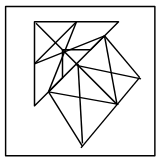a)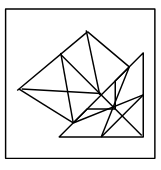b)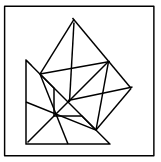c)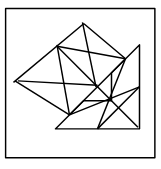d)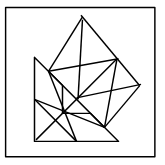Question 10: Find the minor image of the given figure if the mirror is placed at line MN.

a) A

b) B

c) D

d) C

apart from figure ‘C’ ,in all figure arrow either bisect or arranged in different way ,

Hence option ‘B’ is correct.

##### Hence option ‘C’ is correct.

In option A, the image of S is incorrect. In option B, the image of P is incorrect. In option C, the image of J is incorrect. Option D contains the correct image of the given word. Hence, option D is the correct answer.

Option A is the water reflection.

In option C, the mirror image of ‘q’ is incorrect.

In option D, ‘i’ and ‘u’ are interchanged.

Option B is the mirror image.

For getting a water image of something, cut it horizontally to see whether it is showing mirror images of itself or not. If the figure is showing mirror images then it will have the same water image. Here alphabets C and O when cut horizontally will show mirror images of each other so they will have same water image.

The figure given in option b will fit the given figure.

The correct option is ‘a’.

Here, The complete figure will be

Hence, The missing part will be